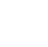# C++ Polymorphism

We already know what polymorphism is in this unit we shall try to understand the two ways in which polymorphism is implemented in C++.

When there are multiple functions with the same name but different parameters then these functions are said to be overloaded. Functions can be overloaded by a change in a number of arguments or/and a change in the type of arguments.

Functions signature refers to the

• return-type of the function.
• data-type of the passed variables
• and finally the number of arguments being passed to the function

``` // C++ program for function overloading #include using namespace std; class overload { public: // function with 1 int parameter void function(int x) { cout << "value of x is " << x << endl; } // function with same name but 1 double parameter void function(double x) { cout << "value of x is " << x << endl; } // function with same name and 2 int parameters void function(int x, int y) { cout << "value of x and y is " << x << ", " << y << endl; } }; int main() { overload obj1; // Which function is called will depend on the parameters passed // The first 'func' is called obj1.func(7); // The second 'func' is called obj1.func(9.132); // The third 'func' is called obj1.func(85,64); return 0; } ```
``` Output: value of x is 7 value of x is 9.132 value of x and y is 85, 64 ```

`In the above example, a single function named function acts differently in three different situations which is the property of polymorphism.`

## `Operator Overloading`

`C++ also offers option to overload operators. For example, we can make the operator (‘+’) for string class to concatenate two strings. We know that this is the addition operator whose task is to add two operands. So a single operator ‘+’ when placed between integer operands , adds them and when placed between string operands, concatenates them.`

``` #include using namespace std; class Complex { private: int real, imag; public: Complex(int r = 0, int i =0) {real = r; imag = i;} // This is automatically called when '+' is used with // between two Complex objects Complex operator + (Complex const &obj) { Complex res; res.real = real + obj.real; res.imag = imag + obj.imag; return res; } void print() { cout << real << " + i" << imag << endl; } }; int main() { Complex c1(10, 5), c2(2, 4); Complex c3 = c1 + c2; // An example call to "operator+" c3.print(); } ```
``` Output: 12 + i9 ```

`In the above example the operator ‘+’ is overloaded. The operator ‘+’ is an addition operator and can add two numbers(integers or floating-point) but here the operator is made to perform addition of two imaginary or complex numbers.`

`Menu`
`HomeCoursesEventsCampus Ambassador Program`
`About`
`About UsPrivacy PolicyTerms and ConditionsCancellation and Refund PolicyContact UsCareers`
`Courses`
`Full-Stack Web Dev Industrial Training Program`
`Data Scientist Industrial Training Program`
`DevTown Bytes`
`RoadmapsCheatsheetsVideosBlogs`
``` ```
``````
```Ask queriesContact Us on WhatsappHi, How Can We Help You? ```